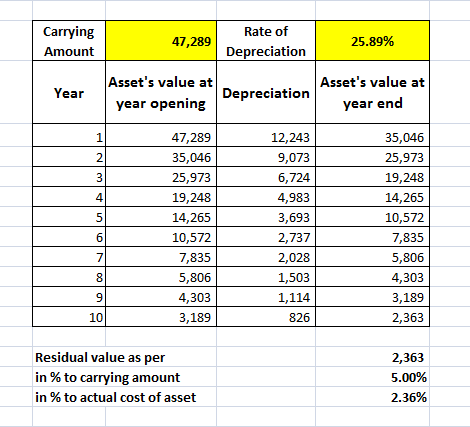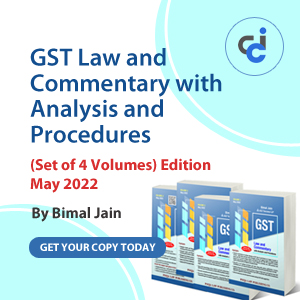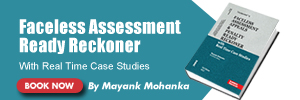# Depreciation, Companies Act 2013 & The Practical Implication

Naresh Kansala , 22 July 2014

As we all know companies bill is passed by both houses of parliaments and some of is section are applicable from 1-4-2014. i.e. out of 470, 283 sections are applicable and section 123 and schedule II is one of them

Before discussing the this, first of all let’s have a look on major changes in schedule II

1. Companies act 1956 does not deal with the amortization of intangible Assets but New Schedule by companies’ act 2014 provide the method to amortize them.

2. Instead of method and rates of Depreciation (whether WDV method or Straight line Method and Single shift or double shift or triple shift) new Act prescribed only assets’ useful life.

3. if a Company, being a class of company specifically prescribed by MCA, can adopt a different useful life longer than what is prescribed in Schedule II, however the same shall be disclosed, as Note on Accounts together with justification. For other companies, useful life cannot be longer than what is prescribed in Schedule II.

4. New act prescribed the residual value to be 5% of cost of asset, in older schedule there is no such prescription as but rates given by schedule are worked out by considering the 5% residual value.

5. New method for double shift and triple shift is prescribed under which addition depreciation of 50% or 100% will be allowed for double and triple shift respectively.

6. The concept of 100% depreciation of assets whose cost is less than Rs. 5000/- is deleted hence under new act it will be depreciated as per other normal provisions of schedule II.

7. Under act if any component of Asset have significant cost and has useful life other than the assets then is should be considered as separate asset for depreciation.

8. List of assets cover is more specific in new schedule.

The Practical Implication:

To apply the schedule II on the running companies schedule II has Note No. 7 which have 2 clauses we’ll discuss them sequentially

Note no. 7(a) from the date this Schedule comes into effect, the carrying amount of the asset as on that date shall be depreciated over the remaining useful life of the asset as per this Schedule II.

Let’s understand with the help if an example:

We taking a general purpose plant and machinery on WDV basis:-

 Sr. No. Particulars Amount/Rate/ Remarks 1 Original cost Rs. 1,00,000/- 2 Useful life and rate of depreciation as per old provisions 20 year and 13.91% 3 Useful life and rate of depreciation as per New provisions 15 years and 18.10% 4 Expired life 5 years 5 Accumulated depreciation for the expired life Rs. 52,711/-

Now i am just pausing here to ask you some questions:-

1. What should be the carrying amount?

2. What should be the remaining useful life of Plant and machinery?

3. What should be the rate of depreciation?

4. What should be the amount for Depreciation?

Now Let’s find out the Answers:

Q.1. What should be the carrying amount?

Ans. Schedule II does not elaborate the meaning of carrying amount so we have to refer AS 28 which defines it as “Carrying amount means the amount at which an asset is recognised in the Balance Sheet after deducting any accumulated Depreciation/amortization and accumulated impairment losses thereon”. This AS does not talk about residual value so we do not deduct any residual value i.e. 5% of original cost of asset to arrive at the carrying amount.

Therefore carrying amount is: original cost less depreciation for the expired life

In given example Column 1 – column 5 i.e. 1,00,000-52,711= 47,289/-

Q2. What should be the remaining useful life of Plant and machinery?

Ans. The remaining useful life of the asset is: Revised life of assets as per schedule II less expired life of asset till 1.4.2014.

In given example: Revised life of Plant and machinery as per schedule II is 15 years and expired life is 5 years therefore remaining useful life is : 15-5= 10years

Q3. What should be the rate of depreciation?

1. Is it on the basis of revised useful life i.e. 15 years as mentioned in schedule II, which comes out 18.10% or,

2. On the basis of remaining useful life which is calculated in Q2. above i.e. 10 years and the rate comes out is 25.89%

Ans. In first instant rate 18.10% seems to be the correct answer, because we are takings about plant and machinery and its rate of depreciation on basis of its revised useful life as mentioned in schedule II is comes out 18.10%.

But as per note 7(a) the asset as on that date shall be depreciated over the remaining useful life of the asset as per this Schedule II.

So we take the rate on the basis of remaining useful life which is 10 years in given example and corresponding rate is 25.89%.

Q4. What should be the amount for depreciation?

Answer: The carrying amount @ rate corresponding to the remaining useful life of asset as at 1st April, 2014

Let's understand it:

If we reduce the residual value from carrying amount then that means we have taken residual value twice for calculating the depreciation because rates that we have derived are already worked out by setting apart 5% residual value. So we do not reduce residual value from carrying amount.

 6 Remaining useful life as at 1st April, 2014 as per new provisions 10 years 7 Carrying amount (1-5) 47,289/- 8 Rate of depreciation on the basis of remaining useful life 25.89%

The result of above is as under:Note 7 (b) From the date this Schedule comes into effect, the carrying amount of the asset as on that date After retaining the residual value, shall be recognised in the opening balance of retained earnings where the remaining useful life of an asset is nil.

Let's understand it with Example:

 Sr. No. Particulars Amount/Rate/ Remarks 1 Original cost Rs. 1,00,000/- 2 Useful life and rate of depreciation as per old provisions 20 year and 13.91% 3 Useful life and rate of depreciation as per New provisions 15 years and 18.10% 4 Expired life 16 years 5 Accumulated depreciation for the expired life Rs. 90,896/- 6 Carrying amount Rs. 9,104/-

Here clarification is needed for residual value that have to be retained, is on the basis of “carrying amount” which comes out as 9104*5%= 455 or on the basis of “cost of asset” which is 100000*5%= 5000

As per my personal views residual value is Rs. 5000/- on the basis of “cost of asset” because schedule II says the residual value should not be more than 5% of cost of the asset.

So the entry in the books of account at the beginning of the year i.e. on 1st April 2014 is

Retained earnings a/c Dr: 4104

To Asset a/c: 4104

(Being the short-fall of Depreciation consequent upon change in the useful Life of Asset provided for after retaining Residual value of 5% and charged against the Opening balance Retained Earnings)

Views and comments are solicited and welcomed even if contrary.

Regards,

Naresh Kansala,

E-Mail: naresh.kansala@gmail.com

Naresh Kansala
(Visit www.nkansala.in for more article)
Category Accounts   Report

40 Likes   1433 Shares   476505 Views

### Popular ArticlesCCI Articles